Test: Progression (AP And GP)- 2

# Test: Progression (AP And GP)- 2

Test Description

## 10 Questions MCQ Test CSAT Preparation for UPSC CSE | Test: Progression (AP And GP)- 2

Test: Progression (AP And GP)- 2 for Banking Exams 2023 is part of CSAT Preparation for UPSC CSE preparation. The Test: Progression (AP And GP)- 2 questions and answers have been prepared according to the Banking Exams exam syllabus.The Test: Progression (AP And GP)- 2 MCQs are made for Banking Exams 2023 Exam. Find important definitions, questions, notes, meanings, examples, exercises, MCQs and online tests for Test: Progression (AP And GP)- 2 below.
Solutions of Test: Progression (AP And GP)- 2 questions in English are available as part of our CSAT Preparation for UPSC CSE for Banking Exams & Test: Progression (AP And GP)- 2 solutions in Hindi for CSAT Preparation for UPSC CSE course. Download more important topics, notes, lectures and mock test series for Banking Exams Exam by signing up for free. Attempt Test: Progression (AP And GP)- 2 | 10 questions in 10 minutes | Mock test for Banking Exams preparation | Free important questions MCQ to study CSAT Preparation for UPSC CSE for Banking Exams Exam | Download free PDF with solutions
 1 Crore+ students have signed up on EduRev. Have you?
Test: Progression (AP And GP)- 2 - Question 1

### Find  the  15th  term  of  an  arithmetic  progression  whose  first  term  is  2  and  the  common  difference is 3

Detailed Solution for Test: Progression (AP And GP)- 2 - Question 1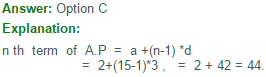Test: Progression (AP And GP)- 2 - Question 2

### What  is  the  sum  of  the  first  15  terms  of  an  A.P  whose  11 th  and   7 th  terms  are  5.25  and  3.25  respectively

Detailed Solution for Test: Progression (AP And GP)- 2 - Question 2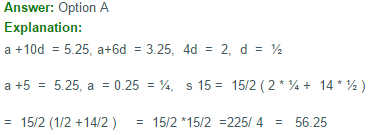Test: Progression (AP And GP)- 2 - Question 3

### If(12+22+32+…..+102)=385,then the value of (22+42+62 + …+202) is :

Detailed Solution for Test: Progression (AP And GP)- 2 - Question 3

(12+22+32+.......102)=385

(22+42+62+.......+202) = 22(12+22+ 32+.....+102 )
=4(385)
=1540

Test: Progression (AP And GP)- 2 - Question 4

In an arithmetic series consisting of 51 terms, the sum of the first three terms is 65 and the sum of the middle three terms is 129. What is the first term and the common difference of the series?

Detailed Solution for Test: Progression (AP And GP)- 2 - Question 4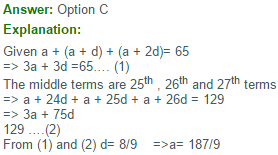Test: Progression (AP And GP)- 2 - Question 5

The sum of the first 100 numbers, 1 to 100 is divisible by

Detailed Solution for Test: Progression (AP And GP)- 2 - Question 5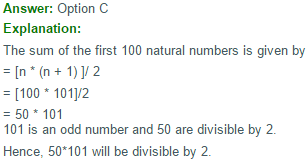Test: Progression (AP And GP)- 2 - Question 6

How many terms are there in G.P 3,6,12,24,….,384?

Detailed Solution for Test: Progression (AP And GP)- 2 - Question 6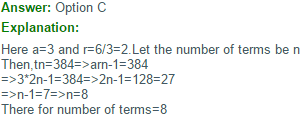Test: Progression (AP And GP)- 2 - Question 7

Four  angles  of  a  quadrilateral  are  in  G.P.  Whose  common  ratio  is  an  intiger.  Two  of  the  angles  are  acute  while  the  other  two  are  obtuse.   The  measure  of  the  smallest   angle  of  the  quadrilateral  is

Detailed Solution for Test: Progression (AP And GP)- 2 - Question 7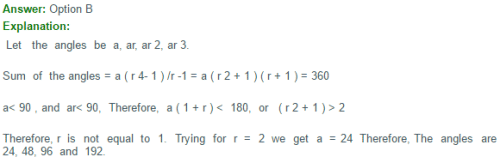Test: Progression (AP And GP)- 2 - Question 8

How many numbers between 11 and 90 divisible by 7?

Detailed Solution for Test: Progression (AP And GP)- 2 - Question 8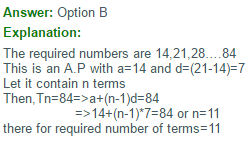Test: Progression (AP And GP)- 2 - Question 9

If Sn denotes the sum of the first n terms in an Arithmetic Progression and S1: S4 = 1: 10

Then the ratio of first term to fourth term is:

Detailed Solution for Test: Progression (AP And GP)- 2 - Question 9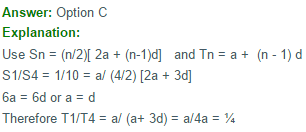Test: Progression (AP And GP)- 2 - Question 10

The sum of the three numbers in A.P is 21 and the product of their extremes is 45. Find the numbers.

Detailed Solution for Test: Progression (AP And GP)- 2 - Question 10

Let the numbers are be a - d, a, a + d
Then a - d + a + a + d = 21
3a = 21
a = 7
and (a - d)(a + d) = 45
a2 - d2 = 45
d2 = 4
d = +2
Hence, the numbers are 5, 7 and 9 when d = 2 and 9, 7 and 5 when d = -2. In both the cases numbers are the same.

## CSAT Preparation for UPSC CSE

72 videos|64 docs|92 tests
 Use Code STAYHOME200 and get INR 200 additional OFF Use Coupon Code
Information about Test: Progression (AP And GP)- 2 Page
In this test you can find the Exam questions for Test: Progression (AP And GP)- 2 solved & explained in the simplest way possible. Besides giving Questions and answers for Test: Progression (AP And GP)- 2, EduRev gives you an ample number of Online tests for practice

## CSAT Preparation for UPSC CSE

72 videos|64 docs|92 tests